## Problem description¶

In this example,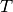tasks must be assigned to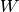workers. Each task has a specific size which represents how much time or effort is required. A worker can have as many tasks as the total size of its tasks is below a fixed bound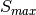. The cost of a task is a function of the worker to which it is assigned.

The aim is to minimize the total cost.

## Naive modelling¶

The problem can be exposed as follows:

Let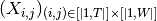be the set of binary variables indicating whether taskhas been assigned to worker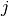. Let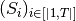be the set of integers representing the size of the tasks. Let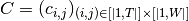with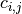the cost associated with the assignement of task. to worker.

The problem is then: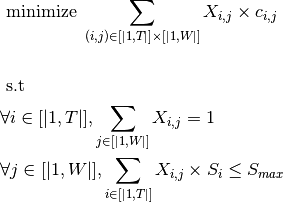This description, despite its ability to be easily understood, is not very effective. Two other more efficient implementations are presented in this section.

## Implementation with standard API¶

Since a a worker can have several tasks assigned to him, it is not possible to use variables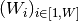giving the task assigned to each worker. The variables have to be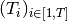with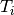the worker assigned to task.

Those variables ensure that each task is assigned once. The only remaining constraint is the capacity constraint on each worker.

A modelling trick is to create an array of variable in which for allthe variableis present as many times as its size. The number of times the number of a worker appears in this array is then equals to the total size of tasks assigned to him and it can be expressed through a cardinality constraint.

This solution can be implemented as follows:

from itertools import chain

def solve(cost_matrix: list, tasks_size: list, worker_size_max: int):
""" Resolution of the assignment with task sizes problem """

# Get the number of tasks and workers from cost_matrix
nb_worker = len(cost_matrix)

try:

# Declaration of the problem
session = KSession()

# Variables declaration

# Initialize variables giving the task assigned to each one of them. Last task is idle.

# Declaration of an array in which the variable of each task is present as many times as its size

# Constraint declaration
# Assert that each worker has a total amount of task's size below worker_size_max by counting how many times it
for worker in range(nb_worker):

# Objective declaration
# Transform the cost matrix into a Kalis object
K_cost_matrix = KIntMatrix(nb_worker, nb_task, 0, "cost_matrix")
for worker in range(nb_worker):

# Objective will be greater than nb_task times the minimum of cost_matrix and lower than the sum of the
# coefficients in cost_matrix
objective_sup = sum(chain(*cost_matrix))

# Get the cost of the tasks given the worker they are assigned to
K_cost_worker, K_elt = {}, {}

0, max([cost_matrix[worker][task] for worker in range(nb_worker)]))

# The objective is the sum of those costs
objective = KIntVar(problem, "cost", int(objective_inf), int(objective_sup))

# Set the objective
problem.setSense(KProblem.Minimize)
problem.setObjective(objective)

# Set the solver
solver = KSolver(problem)

# If the problem is infeasible
if problem.propagate():
# Display various details to help find the source of the problem
problem.display()
problem.printMinimalConflictSet()
print("Problem is infeasible")
exit()

# Launch the optimization
result = solver.optimize()
if result:
solution = problem.getSolution()
# Display the solution
print(f'Total cost : {solution.getValue(objective)}')

# Return the objective
problem.getSolution().display()
return solution.getValue(objective)

except ArtelysException as e:
print(e)

if __name__ == '__main__':
COST = [[90, 76, 75, 70, 50, 74, 12, 68],
[35, 85, 55, 65, 48, 101, 70, 83],
[125, 95, 90, 105, 59, 120, 36, 73],
[45, 110, 95, 115, 104, 83, 37, 71],
[60, 105, 80, 75, 59, 62, 93, 88],
[45, 65, 110, 95, 47, 31, 81, 34],
[38, 51, 107, 41, 69, 99, 115, 48],
[47, 85, 57, 71, 92, 77, 109, 36],
[39, 63, 97, 49, 118, 56, 92, 61],
[47, 101, 71, 60, 88, 109, 52, 90]]

SIZES = [10, 7, 3, 12, 15, 4, 11, 5]
TOTAL_MAX_SIZES = 15

solve(COST, SIZES, TOTAL_MAX_SIZES)


## Implementation with scheduling API¶

Even if the problem does not have a time dimension, it is possible to use the scheduling API to implement it. By considering a time period of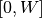, withthe number of workers, a single ressource with a capacity of, andtasks, the statment ‘task i start at j’ can be assimilated to the statment ‘task i has been assigned to worker j’.

By making each task require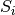unit of capacity from the ressource, it is possible to ensure that the size limit is respected.

A way to implement this method is as follows :

from itertools import chain

def solve(cost_matrix: list, tasks_size: list, worker_size_max: int):
""" Resolution of the task size problem """

# Get the number of tasks and workers from the cost matrix
nb_worker = len(cost_matrix)

try:

# Declaration of the problem
session = KSession()

# Creation of the schedule object with time horizon (0..nb_worker). The start date of a task will then be the
# number of the worker it is allocated to
schedule = KSchedule(problem, "schedule", 0, nb_worker)

# Setting up the resource which are the workers. They are discrete because a worker can take on several task,
# it has a capacity of worker_size_max.
workers = KDiscreteResource(schedule, "workers", worker_size_max)

# Setting up the tasks_array. Each task has a length of 1 - it requires only one worker - and has its on value
# for the workers capacity consumption

# Objective declaration
# Transform the cost matrix into a Kalis object
K_cost_matrix = KIntMatrix(nb_worker, nb_task, 0, "cost")
for worker in range(nb_worker):

# The objective will be greater than nb_task times the minimum of cost_matrix and lower than the sum of the
# coefficients in cost_matrix
objective_sup = sum(chain(*cost_matrix))

# Get the cost of the task given the worker they are assigned to
K_cost_worker, K_elt = {}, {}

0, max([cost_matrix[worker][task] for worker in range(nb_worker)]))

# The objective is the sum of those costs
objective = KIntVar(problem, "cost", int(objective_inf), int(objective_sup))

# Set the objective
schedule.close()
problem.setSense(KProblem.Minimize)
problem.setObjective(objective)

# Set the solver
solver = KSolver(problem)

# If the problem is infeasible
if problem.propagate():
# Display of various details to help find the source of the problem
problem.display()
problem.printMinimalConflictSet()
print("Problem is infeasible")
exit()

# Launch the optimization
result = solver.optimize()
if result:
solution = problem.getSolution()
# Display the solution
print(f'Total cost : {solution.getValue(objective)}')

# Return the objective
problem.getSolution().display()
return solution.getValue(objective)

except ArtelysException as e:
print(e)

if __name__ == '__main__':
COST = [[90, 76, 75, 70, 50, 74, 12, 68],
[35, 85, 55, 65, 48, 101, 70, 83],
[125, 95, 90, 105, 59, 120, 36, 73],
[45, 110, 95, 115, 104, 83, 37, 71],
[60, 105, 80, 75, 59, 62, 93, 88],
[45, 65, 110, 95, 47, 31, 81, 34],
[38, 51, 107, 41, 69, 99, 115, 48],
[47, 85, 57, 71, 92, 77, 109, 36],
[39, 63, 97, 49, 118, 56, 92, 61],
[47, 101, 71, 60, 88, 109, 52, 90]]

SIZES = [10, 7, 3, 12, 15, 4, 11, 5]
TOTAL_MAX_SIZES = 15

solve(COST, SIZES, TOTAL_MAX_SIZES)